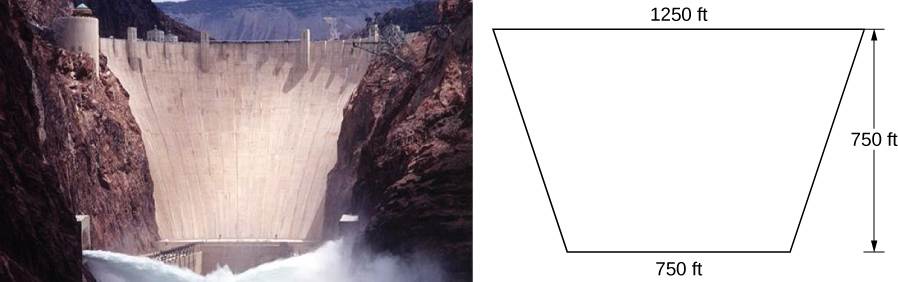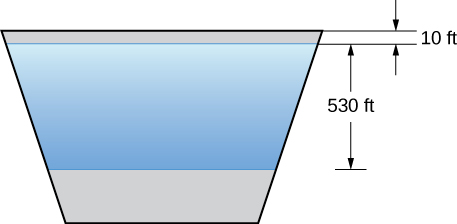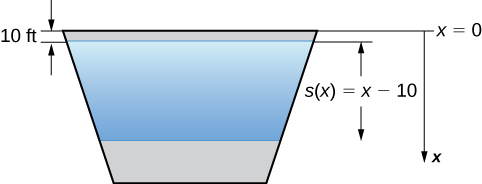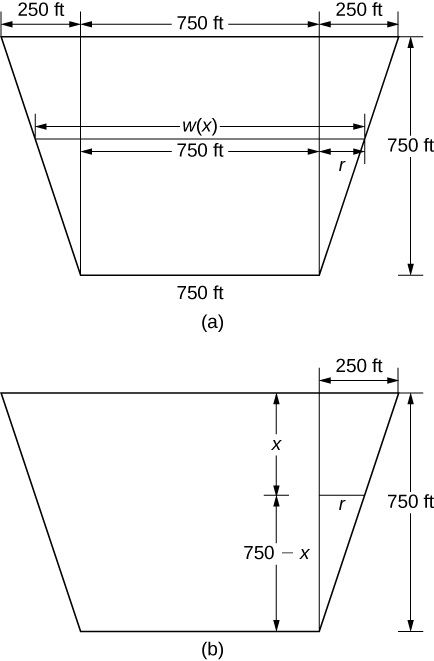## Putting It Together: Applications of Integration

### Finding Hydrostatic Force

We now return our attention to the Hoover Dam, mentioned at the beginning of this chapter. The actual dam is arched, rather than flat, but we are going to make some simplifying assumptions to help us with the calculations. Assume the face of the Hoover Dam is shaped like an isosceles trapezoid with lower base 750 ft, upper base 1250 ft, and height 750 ft (see the following figure).Figure 1.

When the reservoir is full, Lake Mead’s maximum depth is about 530 ft, and the surface of the lake is about 10 ft below the top of the dam (see the following figure).Figure 2. A simplified model of the Hoover Dam with assumed dimensions.

1. Find the force on the face of the dam when the reservoir is full.
2. The southwest United States has been experiencing a drought, and the surface of Lake Mead is about 125 ft below where it would be if the reservoir were full. What is the force on the face of the dam under these circumstances?

1. We begin by establishing a frame of reference. As usual, we choose to orient the $x\text{-axis}$ vertically, with the downward direction being positive. This time, however, we are going to let $x=0$ represent the top of the dam, rather than the surface of the water. When the reservoir is full, the surface of the water is 10 ft below the top of the dam, so $s(x)=x-10$ (see the following figure).Figure 3. We first choose a frame of reference.

To find the width function, we again turn to similar triangles as shown in the figure below.Figure 4. We use similar triangles to determine a function for the width of the dam. (a) Assumed dimensions of the dam; (b) highlighting the similar triangles.

From the figure, we see that $w(x)=750+2r.$ Using properties of similar triangles, we get $r=250-(1\text{/}3)x.$ Thus,

$w(x)=1250-\frac{2}{3}x\text{(step 2).}$

Using a weight-density of 62.4 lb/ft3 (step 3) and applying (Figure), we get

$\begin{array}{cc}\hfill F& ={\displaystyle\int }_{a}^{b}\rho w(x)s(x)dx\hfill \\ & ={\displaystyle\int }_{10}^{540}62.4(1250-\frac{2}{3}x)(x-10)dx=62.4{\displaystyle\int }_{10}^{540}-\frac{2}{3}\left[{x}^{2}-1885x+18750\right]dx\hfill \\ & =-62.4(\frac{2}{3}){\left[\frac{{x}^{3}}{3}-\frac{1885{x}^{2}}{2}+18750x\right]|}_{10}^{540}\approx 8,832,245,000\text{lb}=4,416,122.5\text{t}\text{.}\hfill \end{array}$

Note the change from pounds to tons $(2000$ lb = 1 ton) (step 4). This changes our depth function, $s(x),$ and our limits of integration. We have $s(x)=x-135.$ The lower limit of integration is 135. The upper limit remains 540. Evaluating the integral, we get

$\begin{array}{cc}\hfill F& ={\displaystyle\int }_{a}^{b}\rho w(x)s(x)dx\hfill \\ & ={\displaystyle\int }_{135}^{540}62.4(1250-\frac{2}{3}x)(x-135)dx\hfill \\ & =-62.4(\frac{2}{3}){\displaystyle\int }_{135}^{540}(x-1875)(x-135)dx=-62.4(\frac{2}{3}){\displaystyle\int }_{135}^{540}({x}^{2}-2010x+253125)dx\hfill \\ & =-62.4(\frac{2}{3}){\left[\frac{{x}^{3}}{3}-1005{x}^{2}+253125x\right]|}_{135}^{540}\approx 5,015,230,000\text{lb}=2,507,615\text{t}\text{.}\hfill \end{array}$